• 本代码主要利用MATLAB工具实现MATLAB 矩阵元素的区域访问，简单明了，易于理解
• Matlab矩阵多元素的访问(1)A(a:b:c)表示取数组或矩阵A的第a个元素开始每隔b个步长一直到元素c (2)A([m n l])表示取数组或矩阵A中的第(m,n,l)个元素 (3)A(:,j)表示取A矩阵第j列的所有元素 (4)A(i,:)表示A矩阵第i行...
Matlab矩阵多元素的访问

(1)A(a:b:c)表示取数组或矩阵A的第a个元素开始每隔b个步长一直到元素c
(2)A([m n l])表示取数组或矩阵A中的第(m,n,l)个元素
(3)A(:,j)表示取A矩阵第j列的所有元素
(4)A(i,:)表示A矩阵第i行的全部元素
(5)A(i:i+m,:)表示取A矩阵第i~i+m行的全部元素
(6)A(:,k:k+m)表示取A矩阵第k~k+m列的全部元素
(7)A(i:i+m,k:k+m)表示取A矩阵第i~i+m行内，并在第k~k+m列内的所有元素。
展开全文• 1、普通矩阵的建立  不管是任何矩阵（向量），都可以直接按照行方式输入每个元素：同一行的元素用逗号或者用空格符来分割，且空格个数不限；不同的行用分号分割。所有的元素都处于一方括号[]内。比如：  &gt...
1、普通矩阵的建立

不管是任何矩阵（向量），都可以直接按照行方式输入每个元素：同一行的元素用逗号或者用空格符来分割，且空格个数不限；不同的行用分号分割。所有的元素都处于一方括号[]内。比如：

>>Matrix_2D=[1,2,3;4,5,6;7,8,9]    %生成一个3×3的二维矩阵

用size() 函数可以计算一个矩阵的行数和列数。

2、创建三维数组的三种方法

（1）使用下标创建三维数组

在Matlab命令框中输入以下代码可以创建简单的三维数组：

for i=1:2

for j=1:2

for k=1:2

A(i,j,k)=i+j+k;

end

end

end

（2）使用低维数组创建三维数组

可以先创建一个二维数组，然后再通过第三维数组与其关系生成第三维数组；

D2=[1,2,3;4,5,6;7,8,9];

D3(:,:,1)=D2;

D3(:,:,2)=2*D2;

D3(:,:,3)=3*D2;

(3)使用创建函数创建三维数组

使用cat命令来创建高维数组。cat命令：C=cat(dim,A1,A2,A3,A4......)  其中dim表示的是创建数组的维度，A1,A2,A3,A4表示的是各维度上的数组。

D2=[1,2,3;4,5,6;7,8,9];

C=cat(3,D2,2*D2,3*D2);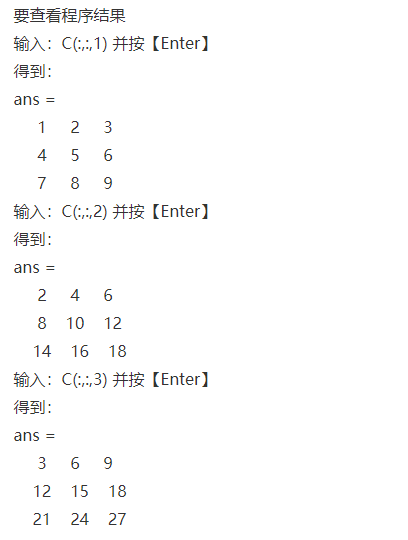3、矩阵的访问

访问矩阵中的元素有两种方式，一种是根据下标访问，另一种是根据序号访问。

以二维矩阵为例，根据下标访问时，将需要访问的矩阵元素的行列坐标写在圆括号内。比如：A(2,3)

可以使用“：”来访问所有坐标，或用end访问末尾坐标；例如：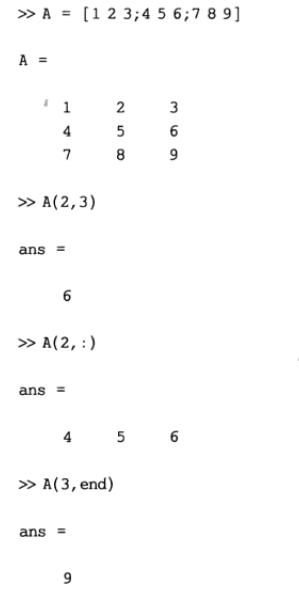矩阵访问一律用()，元胞数组用{}
a(i,j)%第i行第j列队元素
a(:,j)%第j列的所有元素
a(2:end,j)%第j列中第2行到最后一行的元素
a(:,3:5)%第3〜5列的所有元素
a(x)%第x个元素（x为从最左边第1列开始编号，若a为4行5列，则a(10)==a(2,3)）

另一种是根据序号来访问矩阵元素，这种访问方式是将矩阵先转换为一维的向量，然后根据每个元素在该向量中的序号来访问元素，书写时将需要访问的元素序号写在圆括号内即可，如A(10)  ；  也可以使用“：”访问所有或者部分序号，用end访问末尾序号。在MATLAB中所有二维转换为一维的情况都是按照列优先的顺序来访问的。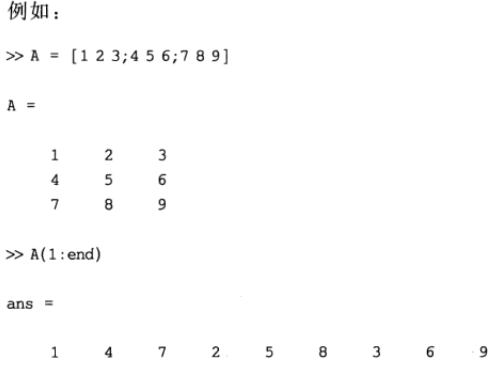两种方式的转换：

sub2ind函数

sub2ind is used to determine the equivalent single index corresponding to a given set of subscript values.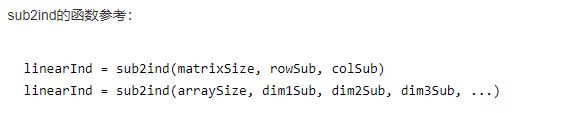二维矩阵： IND = sub2ind(SIZ,I,J)

SIZ是转换矩阵的大小，一般用size函数求得；

I是下标中的行数，J是下标中的列数；

返回值IND是转化后对应的序列号（索引号）；

ind2sub函数

ind2sub和sub2ind这对函数，是互逆的一对函数。ind2sub把数组或者矩阵的线性索引转化为相应的下标；sub2ind则正好相反，将下标转化为线性索引。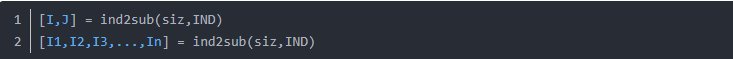展开全文• 欢迎访问我的新博客：http://www.milkcu.com/blog/ ...标题：MATLAB矩阵 内容：MATLAB是MATrix LABoratory的简称，数据的处理是以矩阵为基本单位的。 作者：MilkCu（http://blog.csdn.net/milkcu...


欢迎访问我的新博客：http://www.milkcu.com/blog/
原文地址：http://www.milkcu.com/blog/archives/1370588160.html
标题：MATLAB矩阵
内容：MATLAB是MATrix LABoratory的简称，数据的处理是以矩阵为基本单位的。
作者：MilkCu（http://blog.csdn.net/milkcu）
矩阵的建立
命令窗口直接输入
优点：
灵活构建任意结构、任意数据的矩阵；
语法规则：
矩阵元素应当放在方括号内；行内元素用逗号或空格隔开；行之间用分号或回车分隔；元素可以是数值或表达式；使用“from : step : to”方式
只能生成线性的、等间隔的向量。
当参数step省略时，系统默认step = 1。
使用linspace函数
在指定区间内生成线性等分向量。中间的值，将根据a、b及向量个数n等分产生。
调用格式为linspace(a, b, n)。
其中a表示向量的第一个值，b表示向量的最后一个值，n表示向量中元素的个数。
当参数n省略时，系统默认n = 100。
使用logspace函数
调用格式为logspace(a, b, n)。
其中a表示向量的第一个值以10为底的幂，b表示向量的最后一个值以10为底的幂，n表示向量中元素的个数。
当参数n省略时，系统默认n = 50。
从外部数据文件导入
通过菜单File -> Import Data进行。
特殊矩阵
产生特殊类型矩阵的函数
函数
作用
zeros(m, n)
产生m * n的全0矩阵
ones(m, n)
产生m * n全1矩阵
eye(m, n)
产生m * n单位矩阵
rand(m, n)
产生m * n，以0~1随机矩阵
randn(m, n)
产生m * n，均值为0，方差为1的高斯分布的矩阵
randperm(n)
产生整数1~n的随机排列
magic(n)
产生n阶魔方矩阵
魔方矩阵：每行、列、对角线上的元素和相等。
矩阵下标
全下标方式
第i行j列的元素表示为a(i, j)。
在对元素赋值时，如果i > m或j > n，系统不会报错，并对其余扩充部分以0填充。
单下标方式
矩阵的元素在系统中，仍然以线性方式存储。
用a(x)来表示矩阵的元素。
注意：矩阵元素的读取顺序为“列优先”。
单下标方式a(x)和全下标方式a(i, j)，其下标换算公式为x = (i - 1) * m + j。
子矩阵
a(:, n)表示矩阵a的所有行与第n列，即提取矩阵a第n列元素。a([m n], [i j])表示矩阵第m行与第n行的第i列与第j列。a(m1 : m2, n1 : n2)表示矩阵的第m1行至第m2行的第n1列至第n2列。a(m : end, n)表示矩阵a的第m行至最末行的第n列。a(:, :)表示一个长列矢量，该矢量的元素按矩阵的列进行排列。矩阵的赋值
矩阵赋值方式
赋值方式
使用方法
说明
全下标
a(i, j) = b
给矩阵a的部分元素赋值
单下标
a(s) = b
b为向量，元素个数对应相等
全元素
a( : ) = b
元素总数相等，但行列数可以不同
矩阵元素的删除
利用方括号（[ ]）删除矩阵的某行列，或者它的子矩阵。
矩阵的合并
可以使用方括号（[ ]），将小的矩阵合并成一个大矩阵。
但要注意行列数是否匹配。
矩阵函数
transpose(A)；矩阵A的转置。可以用单引号（'）代替。mean(A)；函数返回行向量，各元素对应A中相应列的算术平均值。mean(A, dim)：当dim = 1时，功能同上；当dim = 2时，返回值为列向量，各元素对应A中相应行的算术平方根。（全文完）

转载于:https://www.cnblogs.com/milkcu/archive/2013/06/07/3808924.html
展开全文• MATLAB矩阵的建立与访问 矩阵访问一律用()，元胞数组用{} a(i,j)%第i行第j列队元素 a(:,j)%第j列的所有元素 a(2:end,j)%第j列中第2行到最后一行的元素 a(:,3:5)%第3〜5列的所有元素 a(x)%第x个元素（x为从最左边第1...
MATLAB矩阵的建立与访问
矩阵访问一律用()，元胞数组用{}
a(i,j)%第i行第j列队元素
a(:,j)%第j列的所有元素
a(2:end,j)%第j列中第2行到最后一行的元素
a(:,3:5)%第3〜5列的所有元素
a(x)%第x个元素（x为从最左边第1列开始编号）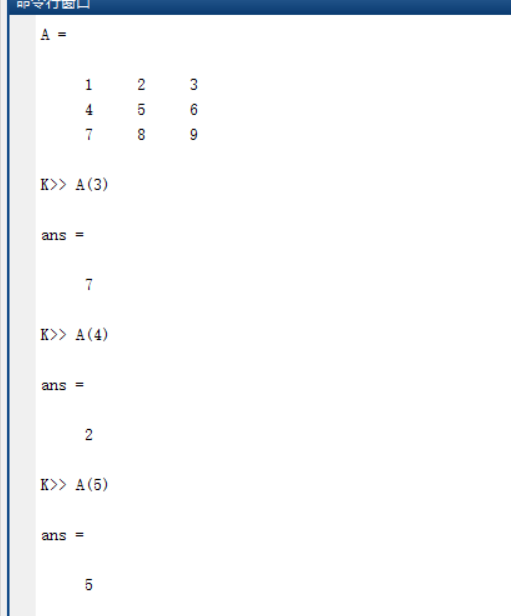展开全文• ## matlab矩阵的集合

千次阅读 2018-01-10 22:08:31
使用元跑数组，来存放矩阵的集合，取值的时候不能使用小括号 a=cell(1,2)%a是一个1*2维的cell数组 赋值时必须使用大括号 >> a(1)='H' Conversion to cell from char is not possible. 无法将char格式转换...
• 本节书摘来自异步社区出版社《MATLAB图像处理超级学习...2.3 MATLAB矩阵寻访 MATLAB图像处理超级学习手册在MATLAB中，矩阵寻访主要有下标寻访、单元素寻访和多元素寻访，下面将对其进行一一介绍。 2.3.1　下标寻访...
• 本节书摘来自异步社区出版社《MATLAB图像处理超级...2.4 MATLAB矩阵的运算 MATLAB图像处理超级学习手册MATLAB中，矩阵的运算包括＋（加）、－（减）、*（乘）、/（右除）、（左除）、^（乘方）等运算。下面对其进行...
• MATLAB矩阵进行多元素访问q=randn(4) q1=q(1,:) %访问第一行 q2=q(1:3,:) %访问1-3行 q3=q(:,2) %访问第二列 q4=q(:) %访问所以元素 运行结果如下
• Matlab中最常用的数据形式莫过于矩阵了，在使用矩阵的过程中我们首先同时也是必须要了解的就是矩阵的储存与访问过程。矩阵的创建一般可以使用以下三种方法：第一种是直接输入矩阵元素；第二种是通过调用函数生成...
• -> Matlab 类型转换器简介：C/C++ 类型（本机、STL、openCV...）和 Matlab 矩阵（编译或运行时）之间的双向转换。 关键词：C、C++、mxArray、OpenCV、IplImage、迭代器、mex、引擎 描述： 考虑以下场景（尤其是在...
• 通过内存映射制作和访问文件。 在处理非常大的数据集/矩阵时非常有用。 您可以只访问其中的特定部分，而不是加载整个矩阵。 非常有效率。
• matlab矩阵自动拼接代码MATLAB，这有什么用？ 撰写者：奥斯汀·博汉农（Austin Bohannon），梅根·沃尔利（Megan Walley），唐纳·汉森（Donner Hanson）和艾莉森·汤普森（Allison Thompson） 介绍 我们选择MATLAB...
• matlab中对矩阵元素的访问： b=1 2 3  4 5 6  11 12 13 单个元素的访问：b(3,2)→12，访问第三行第二列的元素； 整列元素的访问：b(:,3))→[3,6,13]‘，访问了第三列中的所有元素； 整行元素的访问：b...
• 技术凝聚实力 专业创新出版 第4章 MATLAB7.0的矩阵和数组 MATLAB的基本数据结构为矩阵其所有运算都是基于矩阵进行的从形式上看矩阵可以理解成二维的数组矩阵可以方便地存储和访问MATLAB中众多数据类型构成矩阵的元素...
• 矩阵位移法matlab代码F2d：工程师的矩阵刚度分析 F2d是Matlab / Octave中矩阵刚度方法的实现。 与目前的大多数其他实现不同，该实现并不止于节点位移的计算。 F2d还可以开箱即F2d计算内力和反作用力。 对于下游应用...
• 矩阵的操作【访问、赋值、删减、变向、抽取】 矩阵的分块【划分子集】 1.　矩阵的生成 使用 功能1） 特殊字符 [ ] 　直接输入构造数组2） zeros()函数 　构造全0数组 2.　矩阵的扩充 使用 特殊字符 [ ]...
• 本节书摘来自异步社区《MATLAB/Simulink系统仿真超级学习手册》一书中的第2章，第2.3节，作者：MATLAB技术联盟 , 石良臣著，更多章节内容可以访问云栖社区“异步社区”公众号查看 2.3 MATLAB矩阵的运算 MATLAB/...
• ## Matlab数组与矩阵

千次阅读 2017-12-26 22:11:30
数组与矩阵常见操作 该来的，你是逃不掉的。早学晚不学！ 1. 数组的创建与操作clear all % 创建数组示例 A = [ ] B = [6, 5, 3, 2, 1] C = [6 5 3 2 1] D = [6; 3; 4; 3; 2; 1] E = B' %B的转置% 访问数组示例 b1 =...
• 可通过Web界面访问Matrizer。 这是最简单的入门方法。 当前没有文档，但是这些示例提供了关于可行方法的快速指南（有关更多示例，请参见源树的tests /子目录）。 该文件的其余部分描述了如何构建本地命令行版本，...
• 总是习惯c++编程了，惯用对图像三维数据访问顺序为： 列，行，层。表示符号分别是x，y，z 或者i， j，k 三重循环，对于现在的医学三维图像，也多是这样 for(int z = 0; z  for(int y = 0; y  for(int x ...
• matlab矩阵的行列式的代码simple_matrix 这是一个用于矩阵计算的简单库，旨在易于设置和使用。 它包含在一个没有依赖性的文件中，只有一个C ++头文件可以轻松粘贴到任何项目中，并作为头文件包含在内。 目的 没有...
• MATLAB矩阵，单元格或表格作为输入 提供包含您的数据作为输出的LaTeX表的代码 处理数字和文本/字符串数据 轻松包含在您的自动化代码中 轻松直接保存为LaTex文档（请参见runExamples.m中的示例2） 可选功能包括： ...
• 欢迎访问我的博客konosuba.xyz 文章目录运算转置矩阵矩阵标准矩阵乘法元素级乘法复数操作获取尺寸数组索引串联 矩阵与向量的创建在上一篇文章中已经提到，所以这里直接进行操作和运算 运算 现有一矩阵a: >>...数据结构 机器学习
• 'circularBuffer' 类将常规 MATLAB 向量/矩阵转换为圆形向量/矩阵。 在访问/改变 circleBuffer 对象中的元素时，用户指定的索引将被包装成有效的索引。 所有 MATLAB 向量/矩阵运算也适用于 circleBuffer 对象！
• MATLAB利用双下标提取矩阵元素%利用双下标提取矩阵元素 format short r=randn(3) r1=r(1,1) %访问r（1，1）处的值 r2=r(2,3) %访问r（2，3）处的值 r3=r(3,3) %访问r（3，3）处的值 运行结果如下
• 访问矩阵元素 A（2，3） 求矩阵行列 size(A) length(A) 返回行列中最大的那个数 即length（A）=max(size(A)) ndims(A)表示n的维数 矩阵拆分 A(:,1) 表示取第一列 A(1,:)表示取第一行 A=[1 2 3......

# matlab矩阵访问matlab 订阅